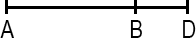## ASVAB Math Knowledge Practice Test 902517

Question 1 of 5
###### Line Segment

A line segment is a portion of a line with a measurable length. The midpoint of a line segment is the point exactly halfway between the endpoints. The midpoint bisects (cuts in half) the line segment.If the length of AB equals the length of BD, point B __________ this line segment.

 bisects intersects midpoints trisects